# Difference between revisions of "Ruled surface"

This article defines a property that makes sense for a surface embedded in$\R^3$, viz three-dimensional Euclidean space. The property is invariant under orthogonal transformations and scaling, i.e., under all similarity transformations.
View other such properties

## Definition

A ruled surface is a surface in Euclidean space$\R^3$ with the property that for any point on the surface, there is a line through that point lying on the surface.

Because, by definition, a surface is connected, an alternative and equivalent definition of ruled surface is as a surface that can be swept by moving a line in space.

### Parametric description

A ruled surface can be described by a parametric description of the form:$\! \mathbf{x}(u,v) = \mathbf{b}(u) + v \delta(u)$

where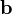$\mathbf{b}$ and$\delta$ are functions that take scalar inputs and give three-dimensional vector outputs. Here, the parameter$u$ controls which line we are on, and the parameter$v$ describes the location of the point on the line. In other words, for every fixed value of$u$, we get a fixed line described with a single parameter$v$. The surface is the union of these lines.

We use the following terminology:

• The line for each fixed value of$u$ is termed a ruling for the surface.
• The function$\mathbf{b}$ is termed the ruled surface directrix or the base curve. For any$u$,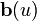$\mathbf{b}(u)$ describes the position of the line.
• The value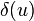$\delta(u)$ describes a direction vector along the line, and the function$\delta$ is termed a director curve.

## Examples

Ruled surface Equational/implicit description Functions$\mathbf{b}$ and$\delta$ in a possible parametric description Comment
Euclidean plane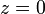$z = 0$ (the$xy$-plane)$\mathbf{b}(u)$ is the vector with coordinates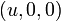$(u,0,0)$ and$\delta(u)$ is the vector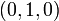$(0,1,0)$. The Euclidean plane is in fact a doubly ruled surface and also a minimal surface.
right circular cylinder (infinite version)$x^2 + y^2 = 1$ (the right circular cylinder with base circle the unit circle in the$xy$-plane and axis along the$z$-axis).$\mathbf{b}(u)$ is the vector with coordinates$(\cos u, \sin u,0)$ and$\delta(u)$ is the vector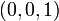$(0,0,1)$.
circular hyperboloid of one sheet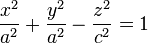$\frac{x^2}{a^2} + \frac{y^2}{a^2} - \frac{z^2}{c^2} = 1$ This is in fact a doubly ruled surface
elliptic hyperboloid of one sheet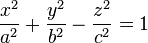$\frac{x^2}{a^2} + \frac{y^2}{b^2} - \frac{z^2}{c^2} = 1$
hyperbolic paraboloid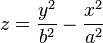$z = \frac{y^2}{b^2} - \frac{x^2}{a^2}$ This is in fact a doubly ruled surface
helicoid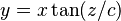$y = x \tan(z/c)$ it is the only ruled minimal surface other than the plane.